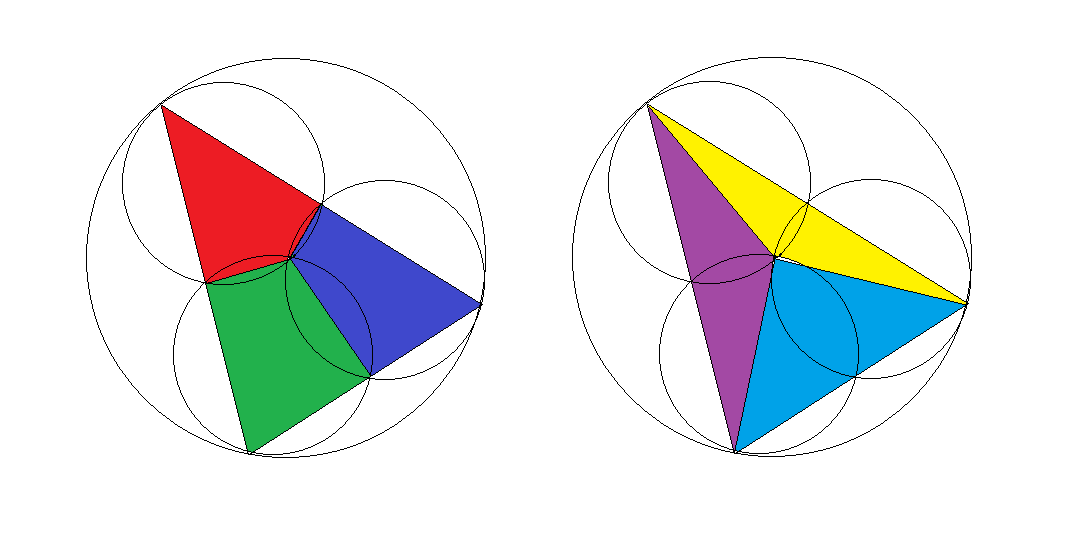# Tangling CirclesThree circles with the same radius intersect at the center of a bigger circle with twice the radius.

Lines are drawn from the intersection points where two small circles meet on the big circle such that they form a triangle, as shown above left. The triangle is then divided into 3 quadrilaterals by drawing the lines from the center of the big circle to the intersection points of the small circles: red, blue, and green regions.

Alternatively, the triangle can be divided into 3 smaller triangles by simply drawing the lines from the center to the vertices: yellow, purple, and cyan regions in the diagram on the right.

Suppose that the ratio of the area of the red region, the area of the dark blue region, and the area of the green region is $11:12:13$.

Then, if the ratio of area of the yellow region, the area of the purple region, and the area of the light blue region is $a:b:c$, where $\gcd(a,b,c) =1$, compute $\frac{a+b+c}{3}$.

×